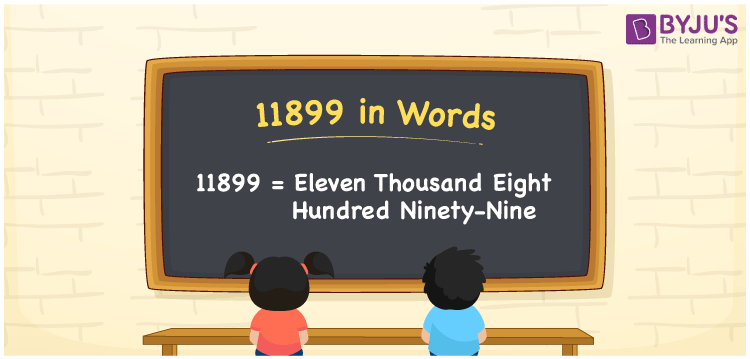# 11899 in Words

We can write 11899 in words as Eleven thousand eight hundred ninety-nine. The word form or the number name of 11899 is Eleven thousand eight hundred ninety-nine. We know that the number name of 11899 is used to express or communicate the number to others. For instance, an amount of Rs. 11899 can be read as “Eleven thousand eight hundred ninety-nine rupees”. Let’s learn how to convert the cardinal number 11899 into words.

 11899 in words Eleven thousand eight hundred ninety-nine Eleven thousand eight hundred ninety-nine in Numbers 11899

## 11899 in English Words

We generally write numbers in words using the English alphabet. So, we spell 11899 in English words as “Eleven thousand eight hundred ninety-nine”.## How to Write 11899 in Words?

11899 is a five-digit number so, let’s create a place value chart with five columns to convert the number 11899 into words. This can be done as given below.

 Ten thousands Thousands Hundreds Tens Ones 1 1 8 9 9

Here, ones = 0, tens = 7, hundreds = 6, thousands = 1, ten thousands = 1

By expanding these digits, we get;

1 × Ten thousand + 1 × Thousand + 8 × Hundred + 9 × Ten + 9 × One

= 1 × 10000 + 1 × 1000 + 8 × 100 + 9 × 10 + 9 × 1

= 10000 + 1000 + 800 + 90 + 9

= Ten Thousand + One thousand + Eight hundred + Ninety + Nine

= Eleven thousand + Eight hundred ninety-nine

= Eleven thousand eight hundred ninety-nine

Therefore, 11899 in words = Eleven thousand eight hundred ninety-nine.

11899 is a natural number that is the successor of 11898 and predecessor of 11900.

11899 in words – Eleven thousand eight hundred ninety-nine

Is 11899 an even number? – No

Is 11899 an odd number? – Yes

Is 11899 a prime number? – No

Is 11899 a composite number? – Yes

Is 11899 a perfect square number? – No

Is 11899 a perfect cube number? – No

## Frequently Asked Questions on 11899 in Words

Q1

### How do you write 11899 in words?

We can write the number 11899 in words as Eleven thousand eight hundred ninety-nine.
Q2

### How to write Rs. 11899 in words on a cheque?

On a cheque, we generally write an amount of Rs. 11899 in words as “Eleven thousand eight hundred ninety-nine rupees only”.
Q3

### Express 11899.75 in words.

We can express the number 11899.75 in words as Eleven thousand eight hundred ninety-nine point seven five.